# Vector operations in two dimensions

### Vector operations in two dimensions

#### Lessons

In this lesson, we will learn:
• How to do addition, subtraction, multiplication and division on vectors in two dimensions
• How to describe the angles of two dimensional vectors
• How to solve for the angle and magnitude of two dimensional vectors with trigonometry.

Notes:

• When adding, subtracting, multiplying, and dividing vectors in two dimensions, the same rules are followed as in one dimensional problems:
• When vectors are added, the vector diagram is drawn by tip-to-tail addition. To add $\vec{A}$ and $\vec{B}$, draw the vector $\vec{A}$, then starting at the tip of $\vec{A}$ draw $\vec{B}$. The tail of $\vec{B}$ connects to the tip of $\vec{A}$.
• Taking the negative of a vector "flips" the vector to point in the opposite direction, while keeping the same magnitude.
• Multiplying or dividing a vector by a positive scalar changes the magnitude of the vector, while keeping the same direction.
• The graphical solution to a vector problem in two dimensions can make a vector triangle that can be solved using trigonometry.
• In this course we will be using navigational notation to specify the angles of vectors, which also called to compass notation when compass directions are used in the problem. Some examples of this notation:
• To specify a displacement of 1 m directly northwest, write 1 m [45° N of W].
• To specify a velocity that that is 1 m/s at an angle of 30° above the ground, write 1 m/s [30° above horizontal]
Right Triangle Trigonometric Equations

$\sin(\theta) = \frac{opp.}{hyp.}$

$\cos(\theta) = \frac{adj.}{hyp.}$

$\tan(\theta) = \frac{opp.}{adj.}$

$a^{2}+b^{2}=c^{2}$ (Pythagorean theorem)

$\theta$: angle, in degrees (°)

$opp.$: side opposite angle

$adj.$: side adjacent angle

$hyp.$: hypotenuse of triangle (longest side, side opposite 90° angle)

$a$ and $b$: non-hypotenuse sides of triangle

$c$: hypotenuse of triangle

• Introduction
Introduction to vector operations in two dimensions

• 1.
Perform tip-to-tail addition in two dimensions

A student arrives at school and from the entrance walks 20 m north to go to English. After, they walk 30 m east to physics class. What is their overall displacement? Answer with a vector diagram and a vector equation that describes the displacements.

• 2.

Solve the following vector equations graphically:

i. $\Delta \vec{d}_{1} + \Delta \vec{d}_{2} = \Delta \vec{d}_{res}$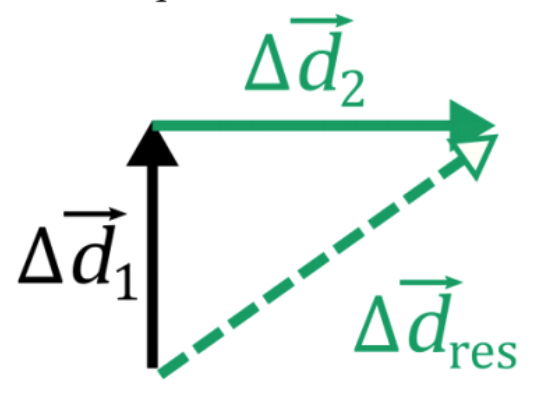ii. $\vec{v}_{1} + \vec{v}_{2} = \vec{v}_{res}$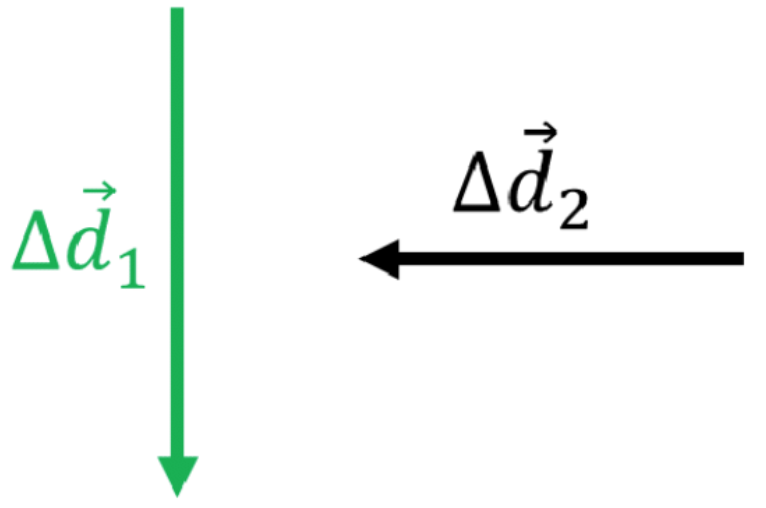iii. $\vec{A} + \vec{B} + \vec{C} = \vec{D}$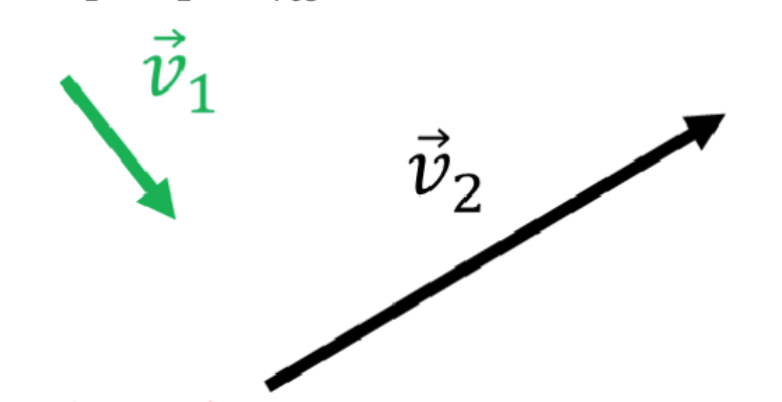• 3.
Solve vector subtraction, multiplication, and division graphically

Solve the following vector equations graphically:

i. $\Delta \vec{d}_{1} - \Delta \vec{d}_{2} = \Delta \vec{d}_{res}$

ii. $2\vec{v}_{1} + 0.2 \vec{v}_{2} = \vec{v}_{res}$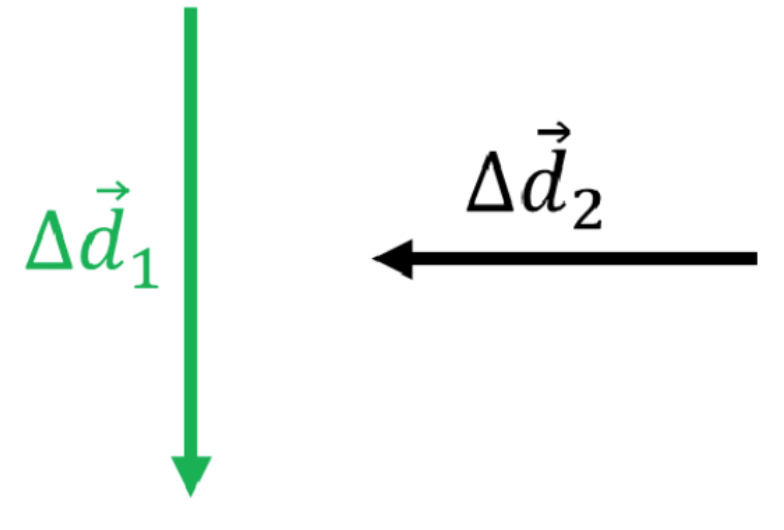iii. $\vec{A} - 2\vec{B} - \frac{\vec{C}}{2} = \vec{D}$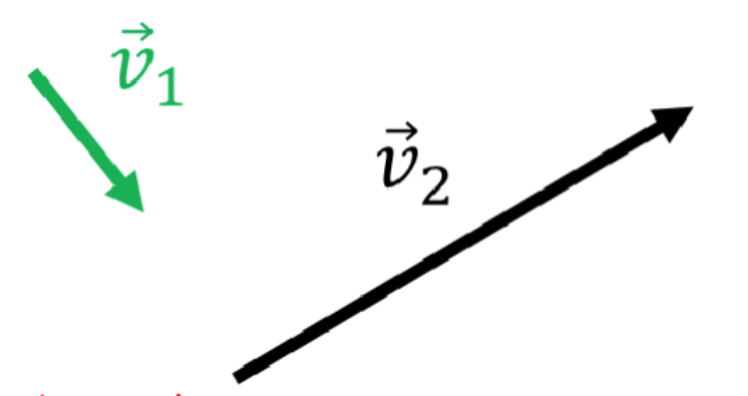iv. $\vec{A} - 2\vec{B} - \frac{\vec{C}}{2} = \vec{D}$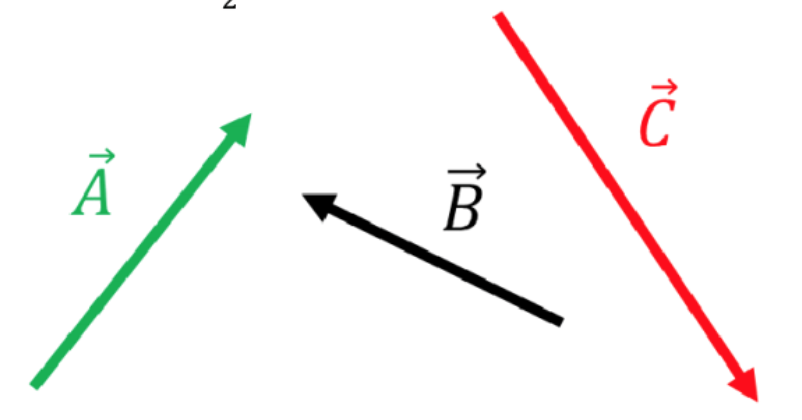• 4.
Write and draw the angles of vectors relative to compass directions

i. Write the vector using vector notation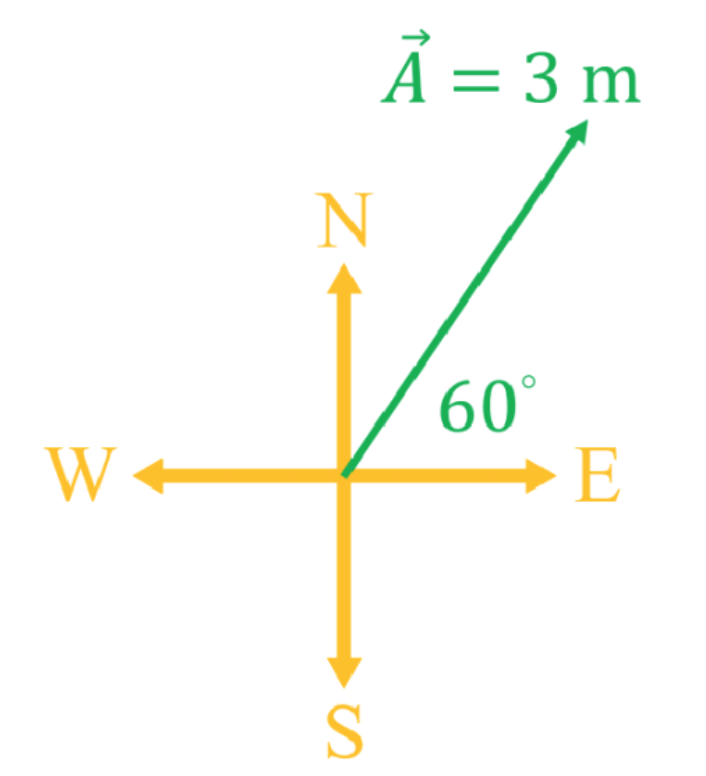ii. Write the vector using vector notation

iii. Draw the vector $\vec{C}$ = 2.5 m [40° S of E] on a set of compass axes.

• 5.
Calculate two dimensional displacement with Trigonometry

A car drives at 13.8 m/s [W] for 115 s. It then turns left and travels south at 19.4 m/s for 135 s. Find the displacement of the car from its starting position.